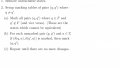# DFA Minimization: Marking Procedure

#### DickCappels

Joined Aug 21, 2008
6,386
For the rest of us, what does "DFA" stand for?

#### zulfi100

Joined Jun 7, 2012
633
Hi,
DFA stands for deterministic finite automata which is drawn in the attached figure (as the first figure) having states q0, q1, q2, q3, q4 and q5. Second figure shows the transition table which shows the movement from one state to another state based upon the inputs 1 or 0. If two different inputs from a state direct the edge to the same state , then its non-deterministic finite automata (NFA) otherwise, its a deterministic finite automata (DFA).

The third figure deals with marking procedure which I want to understand.
Zulfi.

Last edited:

#### WBahn

Joined Mar 31, 2012
25,760
Hi,
DFA stands for deterministic finite automata which is drawn in the attached figure (as the first figure) having states q0, q1, q2, q3, q4 and q5.
You figure doesn't indicate what conditions lead from q5 to q4 and q5 is missing from your table.

It's somewhat moot since there is no way to get to q5, but it is still pretty sloppy and that sloppiness will get you into trouble with this stuff.

Second figure shows the transition table which shows the movement from one state to another state based upon the inputs 1 or 0. If two different inputs from a state direct the edge to the same state , then its non-deterministic finite automata (NFA) otherwise, its a deterministic finite automata (DFA).
You've got it backwards. There's no problem with two different inputs taking the machine from State 1 to State 2 -- that is a deterministic operation. What would make it non-deterministic is if the same input can take the machine from State 1 to two different states.

The third figure deals with marking procedure which I want to understand.
There are many algorithms for minimizing a DFA. I'm not familiar with the one you are trying to use (at least not based on your description this far).

Most of them aim to progressively identify equivalent states, either by breaking states that contain distinguishable subsets or by combining states that are indistinguishable.

#### zulfi100

Joined Jun 7, 2012
633
Hi,
Zulfi.